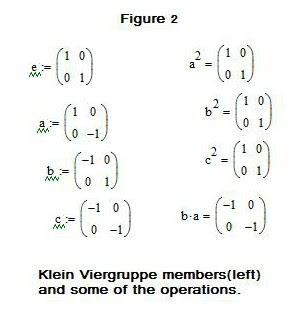## Saturday, May 1, 2010

### Looking at Matrix GroupsIn an earlier blog we examined the fascinating mathematical structures called groups. We recall here the properties that make a group, from the definition given:

A GROUP is a set G with one binary operation defined on it , and G satisfies the axioms:

a) Associative law: a· (b · c) = (a· b) · c
b) Identity element (e): there exists an element e in G such that: e · a = a for all a in G and a · e = a
c) Inverse element: For any a in G there exists an element a^-1 in G such that:
a · a^-1 = a^-1 · a = e

There was also the additional property that defined an Abelian Group:

d) Only if there exists elements a, b in G such that (a · b) = (b · a), then G is said to be an Abelian Group. (Commutative property).

We now want to see how these apply to groups that are posed in matrix form.

A matrix is an assembly of numerical quantities in the form:

(a11 a12)
(a21 a22)

Matrix multiplication, the main operation we will need to know, is easily obtained.

I.e. say the above matrix is denoted A, and another B =

(b11 b12)
(b21 b22)

then A X B =

(a11 a12) (b11 b12)
(a21 a22) (b21 b22)

= [{(a11b11) + (a12b21)} --{(a11 b12)+ (a12 b22)} ]
[(a21b11) + (a22b21) } --{((a21 b12) + (a22 b22)}]

For example, let A=

(1 2)
(1 2)

and B =

(1 3)
(2 2)

then A x B=

(5 7)

(5 7)

The reader is invited to verify this for himself.

We are now in a position to look at some simple 2 x 2 matrix groups, and also assess whether they're Abelian or not.

One example is the special unitary group, SU2. The elements of SU2 are the unitary 2 x 2 matrices with Det = 1 (determinant). [Note: the determinant is taken as follows, using the elements of matrix A, for which Det [A] = (a11 x a22) - (a12 x a21), thus Det [A] = 2 - 2 = 0.]

The elements are shown in their standard matrix form in Fig. 1, and the reader should easily be able to verify that the elements form a group. I tcan be seen, for example, that: II= J*J= J^2 = K*K = K^2 = -i, and IIJ = -JII = K.

Another interesting group is PSL(2, z) which has generators:

s =

(0 1)
(-1 0)

and

t =

(0 -1)
(1 - 1)

Yet another group with 2 x 2 matrix elements is sl(2) which has elements: h, e and f such that:

h =

(1 0)
(0 -1)

e (identity element) =

(0 1)
(0 0)

f =

(0 0)
(1 0)

The elements of the group can easily be shown to obey the relations:

[h, e] = h*e - e*h = 2e, [h.f] = h*f - f*h = -2f, and [e,f] = e*f - f*e = h

(understanding that matrix subtraction simply follows the rule, e.g. :

[A] - [B]

=

{(a11 - b11) (a12 - b12)}
{(a21 - b21) (a22 - b22)}

using the designated elements assigned earlier for the generic matrices A, B)

For the already identified matrices A and B, [A] - [B] =

(0 -1)
(-1 0)

Then there is the famous Klein Viergruppe with members: e (identity), a, b and c. The 2 x 2 matrix members are shown in Fig. 2 along with 4 different operations.

The ambitious reader can gain further insights via the following exercises!

Practice Problems:

1. For the group PSL(2,z) show that the identity element (e) = s^4 = t^3.

2. For the group sl(2) show that:

(a) [h.f] = h*f - f*h = -2f

(b) The "Casimir element", C, of sl(2) is defined according to:

h^2/ 2 + h + 2f*e

find the element

3. Show that the Klein Viergruppe, V4, is Abelian.
Solutions to be given in a future blog!